Next: 3.9 Choice of Long-Wavelength Camera Up: 3 Pre-Observation Preparations Previous: 3.7 Moving Targets

3.8 IUE Aperture Orientation

It is occasionally important to know the astronomical orientation of the spectrograph apertures, as in observations of nebulae, galaxies, and close double stars. Equations for the spacecraft roll angle and the orientation angles of the apertures are given in an article by Schiffer (1980a) and are reprinted below. The orientation, especially with respect to the spectrum, is not always intuitively obvious. As a visual aid in finding the orientation of the apertures, formats of the FES field and the cameras are depicted and discussed below.

Since the FES and spectrographs are fixed within the spacecraft, the FES coordinate system (labelled X and Y) is defined relative to the inertial axes of the spacecraft (see Figure 3.3). These coordinates are used to specify the locations of the apertures, guide stars, and so forth, in the telescope field of view.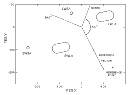Figure 3.3: Aperture plate as viewed by FES No. 2. The locations of the apertures and the reference point are shown as seen by FES No.2. The direction of the reference vector (the direction of stellar motion for a positive pitch slew) is indicated. The X and Y FES coordinates are given in FES fine units (1 unit = 0.26 arcseconds). The North and East directions are shown for a spacecraft roll of 130 degrees.

The aperture and spectrum orientation in the plane of the sky is a function of the spacecraft roll angle. The spacecraft roll is defined as the angle, in the plane of the sky, between north and a reference vector (see below) pointing to the sun. This angle, measured 0 to 360 degrees eastward from the reference vector, changes as a function of the day of the year for a given right ascension and declination, as the spacecraft orientation must keep the solar arrays optimally facing the sun.

Angles in the FES field of view are also defined with respect to the reference vector which points to the sun and is parallel to the pitch direction. When the spacecraft roll is 0 degrees, the reference vector points north. Due to the odd number of reflections in the optical path to the FES, the FES image is displayed in a left-handed coordinate system. All angles in the FES, therefore, increase in a counterclockwise direction. The reference vector for FES No.2, the system in current use (see Figure 3.3), is rotated approximately 28.31 degrees in the FES coordinate system from the negative Y axis toward the negative X axis.

Let the FES position angle in the plane of the sky be defined such that 0 degrees points north and 90 degrees east. [These definitions are slightly different than those of Schiffer (1980a).] The position angle is related to the spacecraft roll by:

position angle = aperture orientation angle - spacecraft roll angle,

where the orientation angles of the apertures are defined with respect to the reference vector and increase counterclockwise from the reference vector. The aperture orientation angles are given in Table 3.2.

 Aperture Orientation Angle Separation LWLA major axis 73° ± 3° -- SWLA major axis 73° ± 2° -- LWLA to LWSA 238° 42.7 arc seconds SWLA to SWSA 242° 39.9 LWLA to SWLA 275° 66.3 LWSA to SWSA 279° 66.2

The orientation of the apertures relative to the FES coordinate system is given in Figure 3.3. The orientation of the field as projected on the camera faceplates may also be determined. The north direction in the FES image is, therefore, rotated counterclockwise from the reference vector by an amount equal to the spacecraft roll angle (see Figure 3.3). Figure 3.4 shows the placement of the large and small apertures with respect to low-dispersion LWP, LWR and SWP spectra. (The apertures have been enlarged for clarity.) While the orientation of the FES coordinate system is different for each camera, the dispersion direction is approximately aligned with the FES Y axis in low dispersion and with the FES X axis in high dispersion.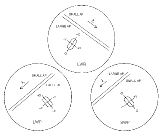Figure 3.4: Aperture orientation on the camera faceplates. The locations of low-dispersion spectra for the large and small apertures are shown for the LWP, LWR, and SWP cameras. The projection of the large aperture is also indicated for each camera. FES No.2 X and Y directions are displayed relative to the apertures and spectra. The relative sizes and angles in this figure are for illustration only.

The spacecraft roll angle can be obtained from GSFC scripts for images after LWR 6528 and SWP 7549, listed as "S/C ROLL". For those scripts where the roll is not given, it may be calculated by the method given below. Note: there is another roll angle ("FSS ROLL") written on the scripts. This is the roll with respect to the sun and is not equivalent to the spacecraft roll angle.

Calculation of the spacecraft roll and Beta angles for a specific date requires the target and solar equatorial coordinates (precessed to 1950.0) and are given by the following equations: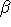= cos¯¹(-sinsin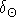- coscoscos(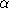-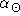))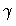= cos¯¹(-sin/(sincos) - tan/tan)

If sin(-) < 0, then S/C roll = 180 +If sin(-) > 0, then S/C roll = 180 -where= Right ascension of the sun= Declination of the sun= Right ascension of the target= Declination of the target.

An example of this calculation forUMa on July 2, 1985 is given below.

The sun's position on July 2, 1985 at 00:00 UT was= 06h41m39.4s,= 23°05'37''(1950)

The targetUMa is at= 13h45m34.3s,= 49°33'44''(1950)

Computed Result: Beta = 97°43'03'', Spacecraft Roll = 63°10'38''.

ThusUMa was in a good Beta angle region for battery power. North is thus 63 degrees counterclockwise from the reference vector. In this case the large apertures are oriented along a line running from NE to SW. For a given camera, the small aperture is located south of the large aperture.

The major axes of the SWLA and LWLA are oriented 10 degrees East of North.

The LWSA is 175 degrees East of North from the LWLA.

The SWSA is 179 degrees East of North from the SWLA.

The SWLA is 32 degrees West of South from the LWLA.Next: 3.9 Choice of Long-Wavelength Camera Up: 3 Pre-Observation Preparations Previous: 3.7 Moving Targets

Last updated: 15 July 1997
jrc